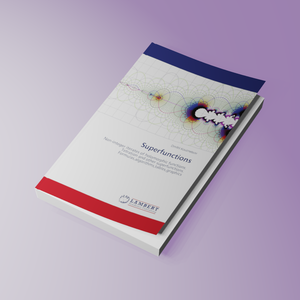Superfunctions

Non-integer iterates of holomorphic functions. Tetration and other superfunctions. Formulas,algorithms,tables,graphics

Regular price
€52,90
Sale price
€52,90
Regular price
Sold out
Unit price
per
Shipping calculated at checkout.

Summary:

Tools for evaluation of superfunctions, abelfunctions and non-integer iterates of holomorphic functions are collected. For a given transferfunction T, the superfunction is solution F of the transfer equation F(z+1)=T(F(z)) . The abelfunction is inverse of F. In particular, superfunctions of factorial, exp, sin are suggested. The Holomorphic extensions of the logistic sequence and those of the Ackermann functions are considered. Among ackermanns, the tetration (mainly to the base b>1) and natural pentation (to base b=e) are presented. The efficient algorithm for the evaluation of superfunctions and abelfunctions are described. The graphics and complex maps are plotted. The possible applications are discussed. Superfunctions significantly extend the set of functions, that can be used in scientific research and technical design. Generators of figures are loaded to http://mizugadro.mydns.jp/BOOK for the free downloading. With these generators, the Readers can reproduce (and modify) the figures from the Book. The Book is intended to be applied and popular. I try to avoid the complicated formulas, but some knowledge of the complex arithmetics and the Cauchy integral should help.

Author:

Dmitrii Kouznetsov

Biographie:

Graduated: Physics Department of the Moscow State University, 1980. Work: USSR, Mexico, USA, Japan. Proven the quantum stability of the optical soliton. Low bound of the quantum noise of nonlinear amplifier. Limit of single mode approximation in Quantum Optics. Theorem on modes of Dirichlet Laplacian. Theory of ridged atomic mirrors. TORI Axioms.

Number of Pages:

328

Book language:

English

Published On:

2020-07-28

ISBN:

978-620-2-67286-3

Publishing House: